# AP Calculus BC Practice Test 45

### Test Information7 questions21 minutes

Calculator Allowed

1. What is the approximate value of y (1) using Euler’s method with a step size of 0.5 and starting at x = 0. If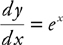and y (0) = 1?

2. If a function f is continuous for all values of x and k is a real number, and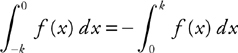, which of the following could be the graph of f?

3. Find the approximate value of y when x = 3.1 if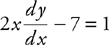and y = 4.5 when x = 3.

4. The velocity of a particle moving on a number line is given by v(t) = sin(t2 + 1), t ≥ 0. At t = 1, the position of the particle is 5. When the velocity of the particle is equal to 0 for the first time, what is the position of the particle?

5. .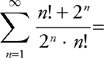6. At what value of x does the graph of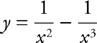have a point of inflection?

7. Twenty ostriches are introduced into a newly built game farm. If the rate of growth of this ostrich population is modeled by the logistic differential equation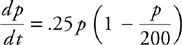with the time t in years, and the farm can support no more than 200 ostriches, how many years, to the nearest integer, will it take for the population to reach 100?